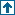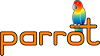parrotcode: Print the Mandelbrot set Contents | Examples

# NAMEexamples/assembly/mandel.pasm - Print the Mandelbrot set

# SYNOPSIS`    % ./parrot examples/assembly/mandel.pasm`

# DESCRIPTIONThis really does something - prints an ASCII-art representation of the Mandelbrot set.

Translated from C code by Glenn Rhoads into Parrot assembler by Leon Brocard <acme@astray.com>.

The C code is:

```    main() {
int x, y, k;
char *b = " .:,;!/>)|&IH%*#";
float r, i, z, Z, t, c, C;
for (y=30; puts(""), C = y*0.1 - 1.5, y--;) {
for (x=0; c = x*0.04 - 2, z=0, Z=0, x++ < 75;) {
for (r=c, i=C, k=0; t = z*z - Z*Z + r, Z = 2*z*Z + i, z=t, k<112; k++)
if (z*z + Z*Z > 10) break;
printf ("%c", b[k%16]);
}
}
}```

We store the following variables in these registers:

```     x,  y, k
I1, I2, I3

r,  i,  z,  Z,  t,  c, C
N1, N2, N3, N4, N5, N6, N7```